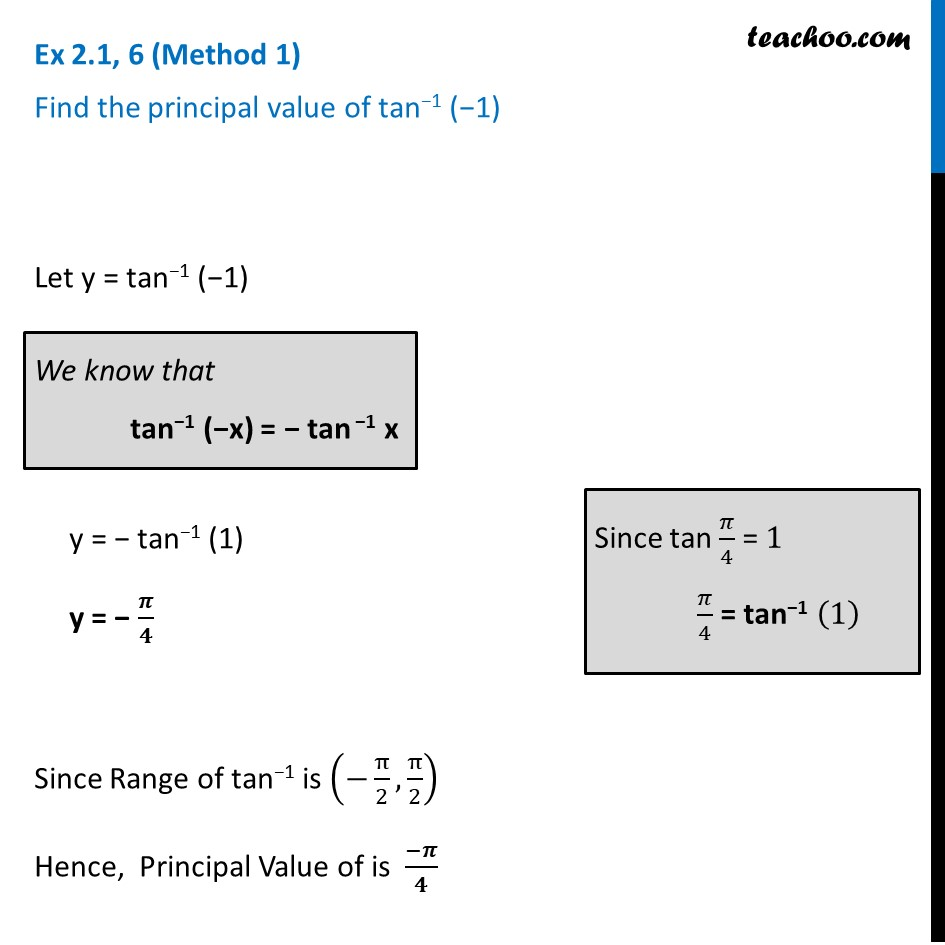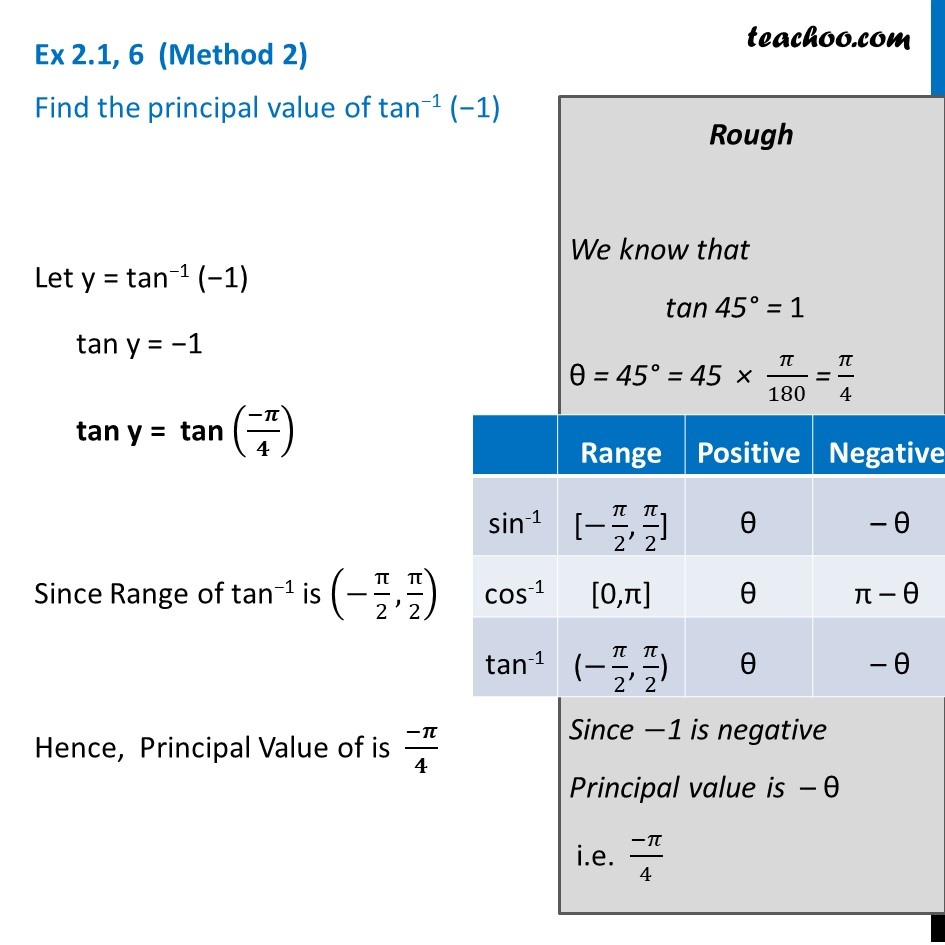Ex 2.1

Chapter 2 Class 12 Inverse Trigonometric Functions
Serial order wiseLearn in your speed, with individual attention - Teachoo Maths 1-on-1 Class

### Transcript

Ex 2.1, 6 (Method 1) Find the principal value of tan−1 (−1) Let y = tan−1 (−1) y = − tan−1 (1) y = − 𝝅/𝟒 Since Range of tan−1 is (−π/2,π/2) Hence, Principal Value of is (−𝝅)/𝟒 We know that tan−1 (−x) = − tan −1 x Since tan 𝜋/4 = 1 𝜋/4 = tan−1 (1) Ex 2.1, 6 (Method 2) Find the principal value of tan−1 (−1) Let y = tan−1 (−1) tan y = −1 tan y = tan ((−𝝅)/𝟒) Since Range of tan−1 is (−π/2,π/2) Hence, Principal Value of is (−𝝅)/𝟒 Rough We know that tan 45° = 1 θ = 45° = 45 × 𝜋/180 = 𝜋/4 Since −1 is negative Principal value is – θ i.e. (−𝜋)/4